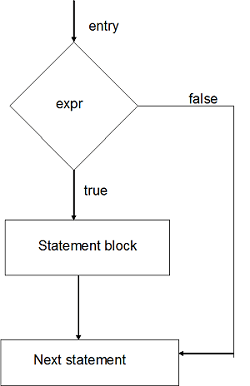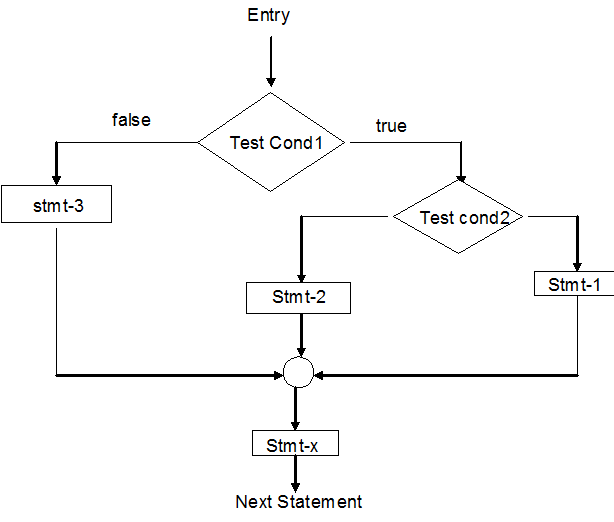# If statement

The 'if' statement is a powerful decision making statement and is used to control the flow of execution of statements. It is basically a two way decision statement and is used in conjunction with as expression .It takes the following form:-
if (test expression )
It allows the computer to evaluate the expression first & then depending on whether the value of the expression (relation(or)condition) is 'true'(non-zero)or 'false'(zero),it transfer the control to a particular statement .This point of program has two paths follow, one for true condition & the other for the false condition.The if statement may be implemented in different forms depending on the complexity of condition to be tested

1. simple if statement
2. if …else statement
3. nested if …else statement

#### 1. Simple if statement:

```                General form:
if (test expression)
{
Statement–block;
}
Statement –x;
```

The statement-block may be a single statement (or) a group of statements. if the test statement is true, the statement-block will be executed, other wise the statement block will be stopped & the execution will be jump to the statement –x.
Note: when the condition is true, both the statement block & the statement-x are executed in sequence.
Flowchart for simple-if:Example: program to find max value of 2 numbers

```                main()
{
int a, b,max;
printf("Enter any 2 values");
scanf("%d%d",&a,&b);
max=a;
if(max< b)
{
max =b;
}
printf("max =%d",max);
getch();
}
```

#### 2)If-else statement

It is an extension of simple if statement.

```                Syntax:
if(test expression)
{
True block statement ;
}
else
{
false block statement;
}
Statement-x;
```

If test expression is true, then the true block statement, immediately following the if statement are executed ; otherwise the false block statement () are executed . In either case , either true-block(or) false block will be executed, not both.Example:To check whether given no is even (or) odd

```                main()
{
int n:
clrscr();
printf("enter any number");
scanf("%d",&n);
if(n%2==0)
printf("even number is even");
else
printf("Given nmber is odd");
getch();
}
```

#### Nested if---else statement:

When a series of decision are involved, we may to have to use more than if-----else statement in nested form as follows:

```                if(test condition)
{
if(test condition)
{
Statement-1;
}
else
{
Statement-2;
}
else
{
Statement-3;
}
Statement-x;
}
```

If the condition 1 is false , the statement-3 will be executed otherwise it continues to perform the second test . IF the condition-2 is true, the statement-1 is evaluated; otherwise statement -2 will be evaluated & then the control is transferred to statement-x.Program to find max of 3 numbers.

```                #include < stdio.h>
void main( )
{
int a,b,c,max;
printf("enter any 3 numbers");
scanf("%d%d%d",&a,&b,&c);
if(a>b)
{
if(a>c)
max=a;
else
max=c;
}
else
{
if(b>c)
max=b;
else
max=c;
}
printf ("max=%d",max);
}
```

There is another way of putting 'if's together when multipath decisions are involved. A multipath decision is a chain of 'if's' in which the stmt associated with each else is an if. It takes the following general form.

```                if(cond1)
Stmt-1;
else if(cond2)
Stmt-2;
else if(cond3)
Stmt-3;
else if(condn)
Stmt-n;
else
Default stmt;
Stmt-x;

```

This construction is known as else if ladder.
The conditions are evaluated from the top to bottom. As soon as true cond is found, the stmt associated with it is executed and the control is transferred to stmt-x(skipping the rest of ladder) when all the conditions become false, then the final else containing the default stmt will be executed.
Example: Write a program to read in the marks of a student and display the grade according to the following information using else-if ladder.

```Average Marks          Grade
80 to 100              A
60 to 79               B
50 to 59               C
40 to 49               D
0  to 39               F(Fail).

```

```              if(marks > 79)
else if(marks > 59)
else if(marks > 49)
else if(marks > 39)
else

```

Program to find maximum of three numbers using else-if ladder.

```              main()
{
int a,b,c,max;
clrscr();
printf("Enter values of a ,b,c");
scanf("%d%d%d",&a,&b,&c);
if(a>b && a>c)
max=a;
else if(b>c)
max=b;
else
max=c;
printf("Maximum is %d",max);
getch();
}
```